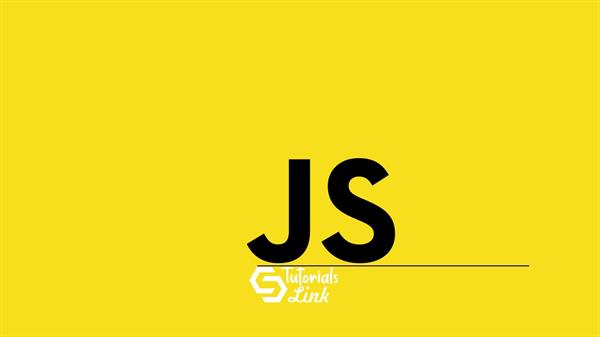Loading, please wait...# What are JavaScript Data Types ( part 2 )

Sep 04, 2020 JavaScript, 4912 Views
JavaScript Data Type part 2

# JavaScript Data Types

In the previous article, you have learned about the strings and dynamic types in JavaScript. Well, it is very much necessary to understand about DataTypes in JavaScript to enlarge idea about datatypes to better understand the way how data are efficiently stored and used to solve the requirement of the purpose. In continuation of the previous article we will now discuss the following data types.

Numbers

Numbers can be stored in JavaScript in various ways be with decimal or without it, lets learn from example.

Example

``````<!DOCTYPE html>
<html>
<body>

<h2>JS Numbers</h2>

<p>NUMBERS CAN BE STORED IN FOLLOWING WAYS:</p>

<p id="TL"></p>

<script>
var A = 99.00;
var B = 99;
var C = 99.99;

document.getElementById("TL").innerHTML =
A + "<br>" + B + "<br>" + C;
</script>

</body>
</html>

``````

Booleans

Sometimes the requirement can be in the form of "True" or "False". Well that being said Javascript provides functionality to store data in form of same. Let's understand from the example below:

``````<!DOCTYPE html>
<html>
<body>

<h2>JS Booleans</h2>

<p>It returns two values: true or false:</p>

<p id="TL"></p>

<script>
var A = 90;
var B = 60;
var C = 90;
document.getElementById("TL").innerHTML =
(A == B) + "<br>" + (B == C)+ "<br>" + (C == A);
</script>

</body>
</html>

``````

ARRAY

The array is an important concept in solving and getting problems solved in a systematic way and efficient way. Let's understand through the following example.

``````<!DOCTYPE html>
<html>
<body>

<h2>JS Arrays</h2>

<p>Array Example.</p>

<p id="tl"></p>

<script>
var cars = ["John","Jennifer","Robin"];

document.getElementById("tl").innerHTML = cars;
</script>

</body>
</html>

``````